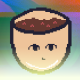# SGDK. Moving a bunch of sprites.

Open the project from the previous lesson, we will finalize it.

First, define the constant, which will specify the array size for sprites. Add it after #include.

``#define CUP_COUNT 10``

Since we will be working with multiple sprites, we will need to create an objectthat will store:

• sprite position
• his speed
• the sprite itself
• sprite size

In general, everything that was stored in individual variables, now, will be placed in the structure from which, we will refer to these variables. This is necessary so that in the future, it would be possible to drive these objects along the cycle.

Replace the old variables with the new structure.

``````typedef struct
{
Sprite *sprite;
s16 posX;
s16 posY;
s16 x_spd;
s16 y_spd;
u16 cup_width;
u16 cup_height;
} Cup;``````

Next line, create an array of these objects (structures).

``Cup cups [CUP_COUNT] ;``

Replace the string SPR_addSprite, before while, with the new variant.

``````s16 i;
for(i = 0; i <CUP_COUNT; i++) {
s16 randSpdX = (random() % 5)+1;
s16 randSpdY = (random() % 5)+1;
Cup oneCup = {NULL, 0, 0, randSpdX, randSpdY, 64, 64};
Sprite* spr = SPR_addSprite(&spr_cup, oneCup.posX,  oneCup.posY, TILE_ATTR(PAL3, 0, FALSE, FALSE));
oneCup.sprite = spr;
cups [i] = oneCup;
}``````

Let’s analyze this code.

``for(i = 0; i<CUP_COUNT; i++) {``

Created a loop that runs through all the elements of the cup array.

``````s16 randSpdX = (random() % 5)+1;
s16 randSpdY = (random() % 5)+1;``````

Random() function – returns a random integer. of this number, took the remainder of the division by 5

``random() % 5``

Thus, we got a number in the range from 0 to 4. We don’t need zero, so add 1.

``Cup oneCup = {NULL, 0, 0, randSpdX, randSpdY, 64, 64};``

We created a oneCup object from a template taken from the Cup structure, now oneCup has its own set of variables. Next, fill the object with values, in the order in which the variables in the structure are arranged. The sprite, until specified, put NULL.

``Sprite* spr = SPR_addSprite(&spr_cup, oneCup.posX, oneCup.posY, TILE_ATTR(PAL3, 0, FALSE, FALSE));``

Add a sprite to the screen, unlike the last lesson, here the coordinates of the oneCup object are used.

``oneCup.sprite = spr;``

Assign this sprite to the object (access to the variables of the object is carried out through a point)

``cups [i] = oneCup;``

And added the object to the array.

Now, let’s start moving these sprites. Change the while loop to the following.

``````while(1)
{
SPR_update();
SYS_doVBlankProcess();
for(i = 0; i <CUP_COUNT; i++) {
Cup oneCup = cups [i] ;
oneCup.posX += oneCup.x_spd;
oneCup.posY += oneCup.y_spd;

if(oneCup.posX > 320-oneCup.cup_width || oneCup.posX < 0)
oneCup.x_spd *= -1;
if(oneCup.posY > 240-oneCup.cup_height || oneCup.posY < 0)
oneCup.y_spd *= -1;
SPR_setPosition(oneCup.sprite, oneCup.posX, oneCup.posY);
cups [i] = oneCup;
}
}``````

Each element of the array.

``for(i = 0; i<CUP_COUNT; i++) {``

We took the object out of the array, and wrote it to a separate variable (for convenience).

``Cup oneCup = cups [i] ;``

After that, they did all the same actions as before, but using the coordinates inside the object.

``````oneCup.posX += oneCup.x_spd;
oneCup.posY += oneCup.y_spd;
if(oneCup.posX > 320-oneCup.cup_width || oneCup.posX < 0)
oneCup.x_spd *= -1;
if(oneCup.posY > 240-oneCup.cup_height || oneCup.posY < 0)
oneCup.y_spd *= -1;
SPR_setPosition(oneCup.sprite, oneCup.posX, oneCup.posY);``````

And wrote the oneCup object back to the array.

Ready. As a result, we got the following.

## Final result.#### bolon667

View all posts by bolon667 →